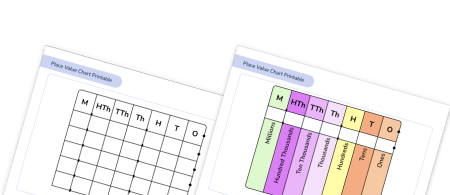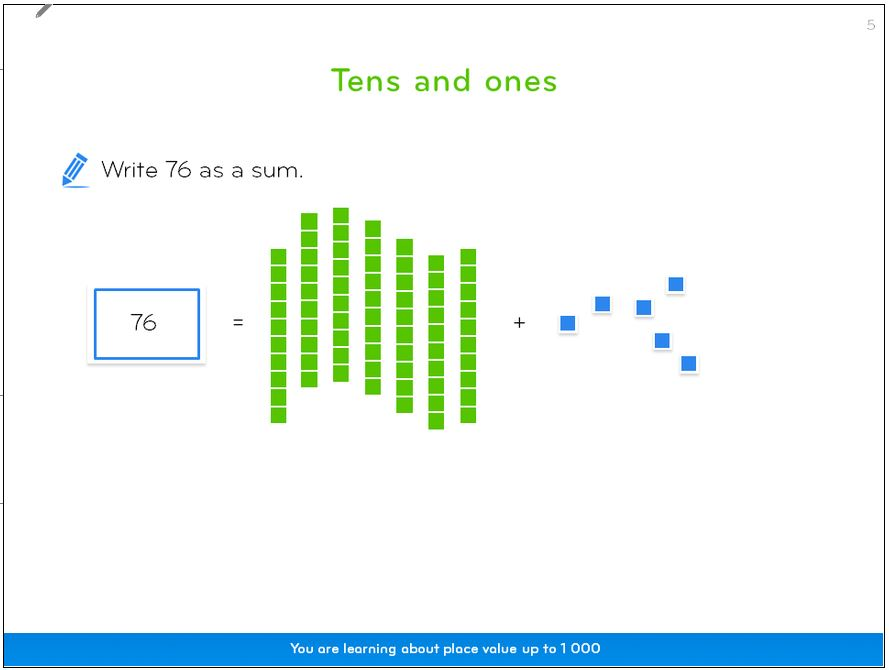Math teaching support you can trustone-on-one tutoring sessionsschools supported

[FREE] Thanksgiving Math Activities

10 fun Thanksgiving math activities for all your elementary students. Includes printable resources!

# What Is Place Value? Explained For Elementary School

In this post we will be explaining what place value is, what it means, and providing you with some questions you can use to test children’s skills!

### What is place value?

Place value is the basis of our entire number system. It is the value of each digit in a number. In other words, the position of a digit in a number determines its value.

For example, the 5 in 350 represents 5 tens, or 50; however, the 5 in 5,006 represents 5 thousands, or 5,000.

It is important that children understand that whilst a digit can be the same, its value depends on where it is in the number.

Children will most likely be taught place value through a chart like this:

Often, these will just appear with letters on them to represent each position: Millions, Hundred Thousands, Ten Thousands, Thousands, Hundreds, Tens, Ones, tenths, hundredths, and so on.

For example, in 27,435, the number 2 is in the ten thousands place and represents 2 ten thousands or 20,000; the number 7 is in the thousands place and represents 7 thousands or 7,000; the number 4 is in the hundreds place and represents 4 hundreds or 400, the number 3 is in the tens place and represents 3 tens or 30; and the number 5 is in the ones place and represents 5 ones or 5.Place Value Chart Printable

Looking for a way to explore place value with your class? Try our blank place value templates of different sizes so students can write directly on them!DOWNLOAD FREE

### When do children learn about place value in school?

Place value is arguably one of the most important areas of the elementary math curriculum.
Each grade level has a set of objectives specifically focused on number and place value. At the start of each school year in elementary school, your child is likely to be reviewing previous place value work and building on it with larger (or smaller in the case of decimals) numbers.An example ‘stepping stone’ from using manipulatives – using pictorial representations of them – taken from Third Space Learning’s online maths lessons.

#### Schools following Common Core:

In kindergarten, students should:

• Count to 100 by ones and by tens.
• Count forward from any given number.
• Write numbers to 20 in numerals.

Students may also begin to recognize place value in numbers beyond 20 by reading, writing, counting and comparing numbers up to 100, supported by objects and visual representations.

• Recognize the place value of each digit in a two-digit number (tens, ones).
• Read and write numbers to at least 100 in numerals and in words.

By the end of 1st grade, students should know the number bonds to 20 and be precise in using and understanding place value.

• Recognize the place value of each digit in a three-digit number (hundreds, tens, ones).
• Count within 1000; skip-count by 5s, 10s, and 100s.
• Read and write numbers up to 1000 using numerals, number names, and expanded form.

• Use place value understanding to round whole numbers to the nearest 10 or 100.

• Recognize that in a multi-digit whole number, a digit in one place represents ten times what it represents in the place to its right.
• Read and write multi-digit whole numbers using base-ten numerals, number names, and expanded form.
• Use place value understanding to round multi-digit whole numbers to any place.

• Recognize that in a multi-digit number, a digit in one place represents 10 times as much as it represents in the place to its right and 1/10 of what it represents in the place to its left.
• Read, write, and compare decimals to thousandths.
• Use place value understanding to round decimals to any place.

#### Other schools:

Other schools not following Common Core follow the same progression of concepts for teaching place value as shown above, although some individual standards may vary slightly.

### How are children be taught place value at elementary school?

Throughout elementary school, two concrete math resources are used to help make place value easy  for children to understand. Both of the following aids do this by representing it in a visual manner.

#### Base ten blocks

Base ten blocks  (also known as “base 10 blocks”) are cubes that are used to represent units/ones, tens, hundreds, and thousands.

These cubes are a simple way for children to make different numbers. To make the number 174, they would need to gather one set of 100, 7 sets of 10, and four single cubes.

#### Ten frames

Ten frames are rectangular grids with two rows of five boxes. They are commonly used in primary classrooms to represent numbers within the context of “ten.” This helps students build a strong number sense by providing students with simple, visual representations of ones and tens.

To use a ten frame, students are given counters which they can manipulate within the ten frame to create different numbers and learn about place value. They can also use two ten frames – a full ten frame to show a ten, and then a second ten frame to show the ones – to create numbers 11-20. Ten frames are also used when students are just beginning to learn how to add and subtract.

Understanding place value is crucial for children before they can move on to adding and subtracting two-digit numbers.

If you’re interested in digging deeper into this topic, then we recommend reading this detailed guide on teaching place value and trying out some of these place value games.

### How does place value relate to other areas of math?

Place value is intrinsically linked to many other areas of math; a solid understanding of it is vital in order to allow children to competently add, subtract, multiply, and divide, among other things.

### Place value and other areas of math within each grade level

#### Schools following Common Core:

In Kindergarten, students will compose (make) and decompose (take apart) numbers from 11 to 19 into tens and ones by using objects or drawings.

In 1st grade, students will use place value and number facts to add and subtract within 100.

In 2nd grade, students will add and subtract within 1000 using strategies based on place value, properties of operations, and/or the relationship between addition and subtraction, and they will explain why these strategies work.

In 3rd grade, place value is used in addition and multiplication. Students will add and subtract within 1000 using strategies based on place value, properties of operations, and/or the relationship between addition and subtraction.

Students will also multiply one-digit whole numbers by multiples of 10 in the range 10-90 using strategies based on place value and properties of operations.

In measurement, 3rd grade students build on their understanding of place value by relating addition and multiplication to concepts of area and perimeter.

In 4th grade, students will add and subtract multi-digit whole numbers using the standard algorithm.

Students will also multiply a whole number of up to four digits by a one-digit whole number, multiply two two-digit numbers, and divide up to four-digit dividends and one-digit divisors, all using strategies based on place value.

In measurement, students will use their knowledge of place value and the four operations to solve word problems involving distances, intervals of time, liquid volumes, masses of objects, and money. They will also use multiplication and division to convert between units such as meters and kilometers.

In 5th grade, students will multiply multi-digit whole numbers using the standard algorithm and divide whole numbers with up to four-digit dividends and two-digit divisors

Students will use their place value understanding to add, subtract, multiply, and divide decimals to hundredths.

In measurement, students will convert among different-sized standard measurement units within a given measurement system.

#### Other schools:

Other schools not following Common Core still follow the same progression of concepts as shown above; students begin in Kindergarten composing and decomposing tens and ones and work their way through the four operations until finally, in 5th grade, they are using the four operations involving decimals. Some standards may vary by state.

### Place value practice questions for elementary school children

Try these to get an idea of how comfortable your child is with the place value system.

1) In the following numbers, what is the value of the digit 7? a) 405.7   b) 30,070

2) Jack has four number cards: 2, 3, 4 and 7. He uses each card once to make a four-digit number. He places 4 in the tens column; 2 so that it has a higher value than any of the other digits, and the remaining digits so that 7 has the higher value. What number did Jack make?

3) Look at this number: 23,451.96. Write the digit that is in the   a) hundreds place b) hundredths place.

4) Write the number 402,037 in words.

This blog is part of our series of blogs designed for teachers, schools and parents supporting home learning.

For other math terminology, check out our free elementary math dictionary for kids has parent and child-friendly definitions of all key math vocabulary.

Do you have students who need extra support in math?
Give your students more opportunities to consolidate learning and practice skills through personalized math tutoring with their own dedicated online math tutor.

Each student receives differentiated instruction designed to close their individual learning gaps, and scaffolded learning ensures every student learns at the right pace. Lessons are aligned with your state’s standards and assessments, plus you’ll receive regular reports every step of the way.

Personalized one-on-one math tutoring programs are available for:

The content in this article was originally written by primary school teacher Sophie Bartlett and has since been revised and adapted for US schools by elementary math teacher Katie Keeton.##### Sophie Bartlett
Year 6 Teacher
Sophie teaches mixed age classes at a small school in central England. She is a self confessed grammar pedant and number nerd so we've welcomed her as a regular author and editor for Third Space Learning.
x#### Place Value Chart Printable

Looking for a way to explore place value with your class? Try our blank place value templates of different sizes so students can write directly on them!

#### Place Value Chart PrintableLooking for a way to explore place value with your class? Try our blank place value templates of different sizes so students can write directly on them!